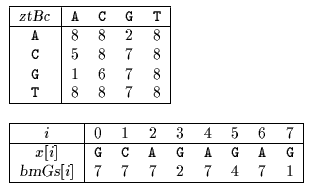Name
• String
• Substring
• Search
Edit

Zhu and Takaoka designed an algorithm which performs the shift by considering the bad-character shift (see chapter Boyer-Moore algorithm) for two consecutive text characters.

During the searching phase the comparisons are performed from right to left and when the window is positioned on the text factor y[j .. j+m-1] and a mismatch occurs between x[m-k] and y[j+m-k]while x[m-k+1 .. m-1]=y[j+m-k+1 .. j+m-1] the shift is performed with the bad-character shift for text characters y[j+m-2] and y[j+m-1]. The good-suffix shift table is also used to compute the shifts.

The preprocessing phase of the algorithm consists in computing for each pair of characters (ab) with ab inthe rightmost occurrence of ab in x[0 .. m-2].

For a,b inztBc[ab]=k iffk
ork=m-1 and x=b and ab does not occur in x[0 .. m-2]
ork=m and xb and ab does not occur in x[0 .. m-2]

It also consists in computing the table bmGs (see chapter Boyer-Moore algorithm).

The preprocessing phase is in O(m+2) time and space complexity. The searching phase has a quadratic worst case.

Main features:

• variant of the Boyer-Moore algorithm;
• uses two consecutive text characters to compute the bad-character shift;
• preprocessing phase in O(m+2) time and space complexity;
• searching phase in O(mn) time complexity.

Example:

Preprocessing phaseztBc and bmGs tables used by Zhu-Takaoka algorithm.

Searching phase

 G C A T C G C A G A G A G T A T A C A G T A C G 1 G C A G A G A G

Shift by: 5 (ztBc[C][A])

 G C A T C G C A G A G A G T A T A C A G T A C G 8 7 6 5 4 3 2 1 G C A G A G A G

Shift by: 7 (bmGs)

 G C A T C G C A G A G A G T A T A C A G T A C G 3 2 1 G C A G A G A G

Shift by: 7 (bmGs)

The Zhu-Takaoka algorithm performs 12 character comparisons on the example.

C